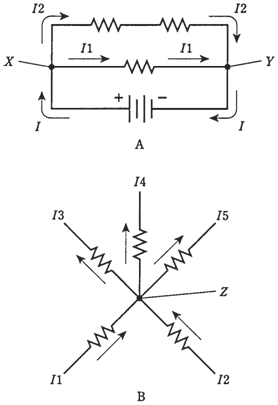## Kirchhoff’s first law Assignment Help

Assignment Help: >> Electrical Engineering - Kirchhoff’s first law

Kirchhoff's first law

The physicist named Gustav Robert Kirchhoff was a researcher and experimentalist in electricity back in time before radio, before electric lighting, and before much was understood about how the currents flow.

Kirchhoff reasoned that current should work something like water in a network of pipes, and that the current going into any point has to be same as the current going out. This is true for the any point in a circuit, no matter how many branches lead in or out of the point. Two examples are shown in the Figure given below.Figure: Kirchhoffs First Law. At A, the current into either X or Y are same as the current out of that point:

I = Il + I2. At the point B, the current into Z equals the current out of Z: Il + I2 = I3 + I4+ I5.

In a network of water pipes which does not leak, and into which no water is added along the way, the total number of cubic feet going in has to be the same as the total volume going out. Water cannot form from nothing, nor does it disappear, inside a closed system of pipes. Charge carriers, thought Kirchhoff, should act the same way in an electric circuit.

This is Kirchhoff's First Law states that an alternative name might be of the law of conservation of current.

Problem: 1

Suppose all the 3 resistors have values of 100 Ω, and that I1=2.0 A while I2 = 1.0 A. What do you mean by battery voltage?

First, find the current I drawn from battery. It should be 3.0 A; I=I1+I2 = 2.0+1.0=3.0  Next, find resistance of the whole combination. The two resistors  of100-Ω in series give a value of 200 Ω, and this is in parallel with the 100 Ω. You can perform the calculations and find the total resistance, R, across the battery, E, is 66.67 Ω. Then E = IR =66.67*3.0=200 volts.